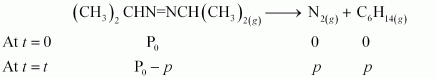# 4.20   For the decomposition of azoisopropane to hexane and nitrogen at 543K, the following data are obtained.Calculate the rate constant.

M manish

Decompostion is represented by equation-After t time, the total pressure

So,

thus,

for first order reaction,

now putting the values of pressures,

when t =360sec

when t = 270sec

So,

Exams
Articles
Questions